update：题目地址：here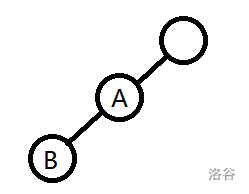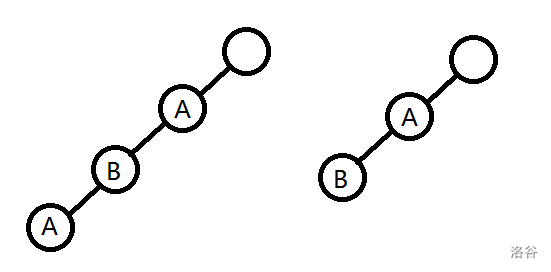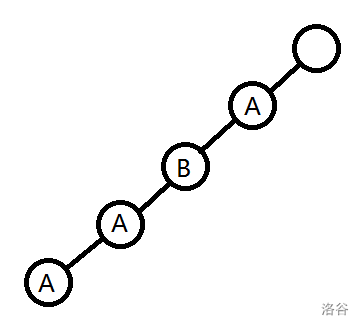$f_i$表示已经有第 $i$种字符的可以作为结尾节点的总数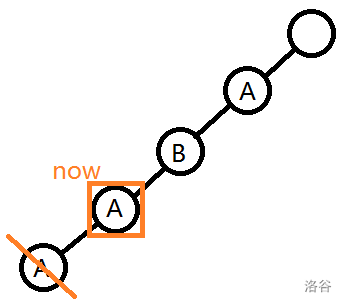P.S. 看到这个题的模数有点懵，太菜了不知道是什么，于是……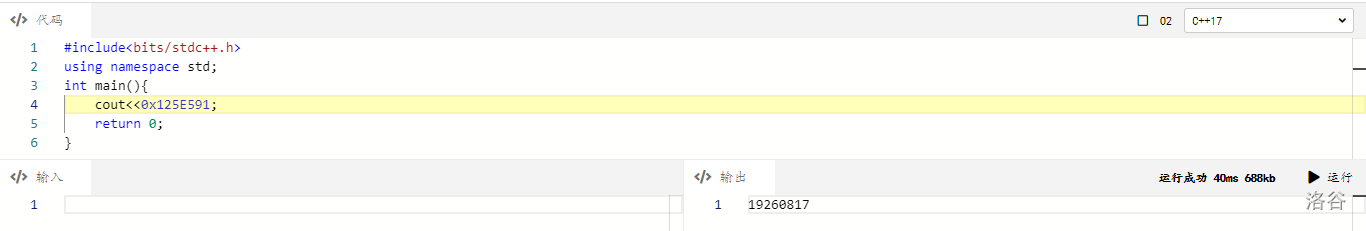#include<bits/stdc++.h>
using namespace std;
const int yyf=19260817;//日膜 yyf
int n,m;
int f;
char s;
int main(){
cin>>n>>m;
scanf("%s",s+1);
char ch;int ans=1;
while(m--){
cin>>ch;
if(ch=='u'){                            //删除操作
if(!n)continue;                     //没有可以删除的了
else{
ans=(ans+1)%yyf;                //+1
f[s[n]-'A']=(f[s[n]-'A']+1)%yyf;//+1
--n;                            //删除节点往上跳
}
}
else{
int tmp=f[ch-'A'];                  //暂时保存
f[ch-'A']=ans;                      //赋值
ans=((ans+ans-tmp)%yyf+yyf)%yyf;    //算 ans
}
}
cout<<ans;
return 0;
}